Like   Tweet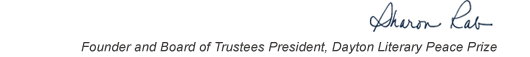table div table+table+table+table div table{width:100%;padding:0}table div table+table+table+table div table img{width:96.23%;padding:0;float:none}table div table+table+table+table div table td{width:100%;padding:0 1.88% 18px}/* styles */This list includes the winners and runners-up for 2007 and we have rich background information on each one on our website.

▪ Under Past Winners. scroll down to the year and click on it.
▪ If you click on the winners and runners-up, you will find the author’s bio, the judges’ citation, and the writer’s reflection on literature and peace.
 ▪ Under Past Winners. scroll down to the year and click on it.
 ▪ If you click on the winners and runners-up, you will find the author’s bio, the judges’ citation, and the writer’s reflection on literature and peace.

On the bottom right of the home page, you can click on COREScholar, which is built by the Chair of our Curriculum Committee Carol Loranger, Associate Dean of the College of Liberal Arts at Wright State, and maintained for us by Wright State University. There you can find interviews, TED Talks, articles, other books, films, etc. by and about each of our winning and runner-up authors. It is a great resource for students, book clubs, and readers who would like to explore an author in depth.

 table div table+table+table+table+table+table div table{width:100%;padding:0}table div table+table+table+table+table+table div table img{width:96.23%;padding:0;float:none}table div table+table+table+table+table+table div table td{width:100%;padding:0 1.88% 18px}/* styles *//* styles */ “Read global; buy local.” — Marlon James, DLPP 2009 Fiction Winner for The Book of Night Women
 table div table+table+table+table+table+table+table+table div table{width:100%;padding:0}table div table+table+table+table+table+table+table+table div table img{width:96.23%;padding:0;float:none}table div table+table+table+table+table+table+table+table div table td{width:100%;padding:0 1.88% 18px}/* styles */## 2007 Finalists - Fiction

 table div table+table+table+table+table+table+table+table+table+table div table{width:100%;padding:0}table div table+table+table+table+table+table+table+table+table+table div table img{width:96.23%;padding:0;float:none}table div table+table+table+table+table+table+table+table+table+table div table td{width:100%;padding:0 1.88% 18px}/* styles */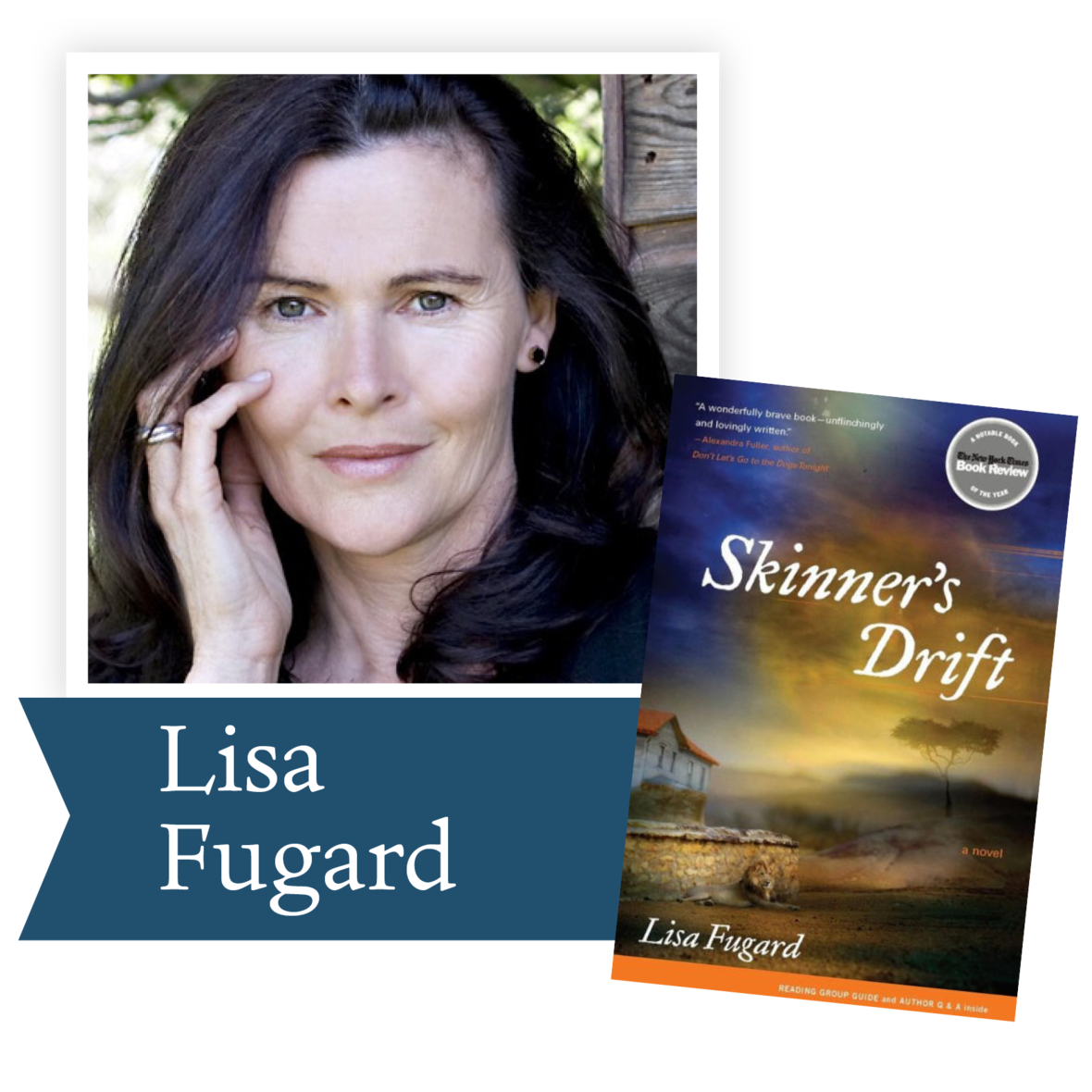Skinner’s Drift by Lisa Fugard (Scribner): In this beautiful, brave, and extraordinarily moving first novel, Lisa Fugard paints a haunting portrait of a young woman coming to terms with her family's violent past as her homeland, South Africa, confronts its own bloody history.

 table div table+table+table+table+table+table+table+table+table+table+table+table div table{width:100%;padding:0}table div table+table+table+table+table+table+table+table+table+table+table+table div table img{width:96.23%;padding:0;float:none}table div table+table+table+table+table+table+table+table+table+table+table+table div table td{width:100%;padding:0 1.88% 18px}/* styles */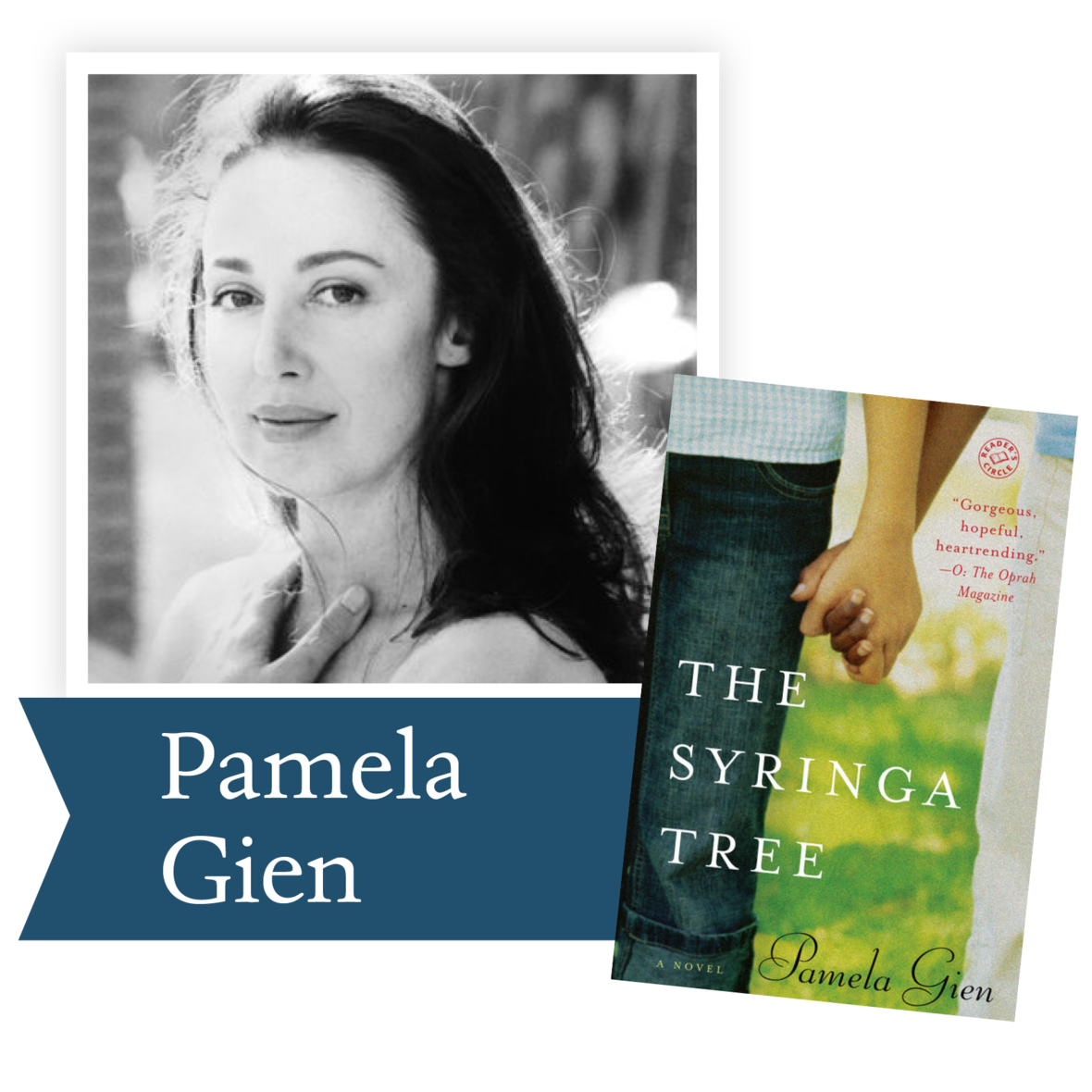The Syringa Tree by Pamela Gien (Random House): In this heartrending and inspiring novel set against the gorgeous, vast landscape of South Africa under apartheid, award-winning playwright Pamela Gien tells the story of two families–one black, one white–separated by racism, connected by love.

 table div table+table+table+table+table+table+table+table+table+table+table+table+table+table div table{width:100%;padding:0}table div table+table+table+table+table+table+table+table+table+table+table+table+table+table div table img{width:96.23%;padding:0;float:none}table div table+table+table+table+table+table+table+table+table+table+table+table+table+table div table td{width:100%;padding:0 1.88% 18px}/* styles */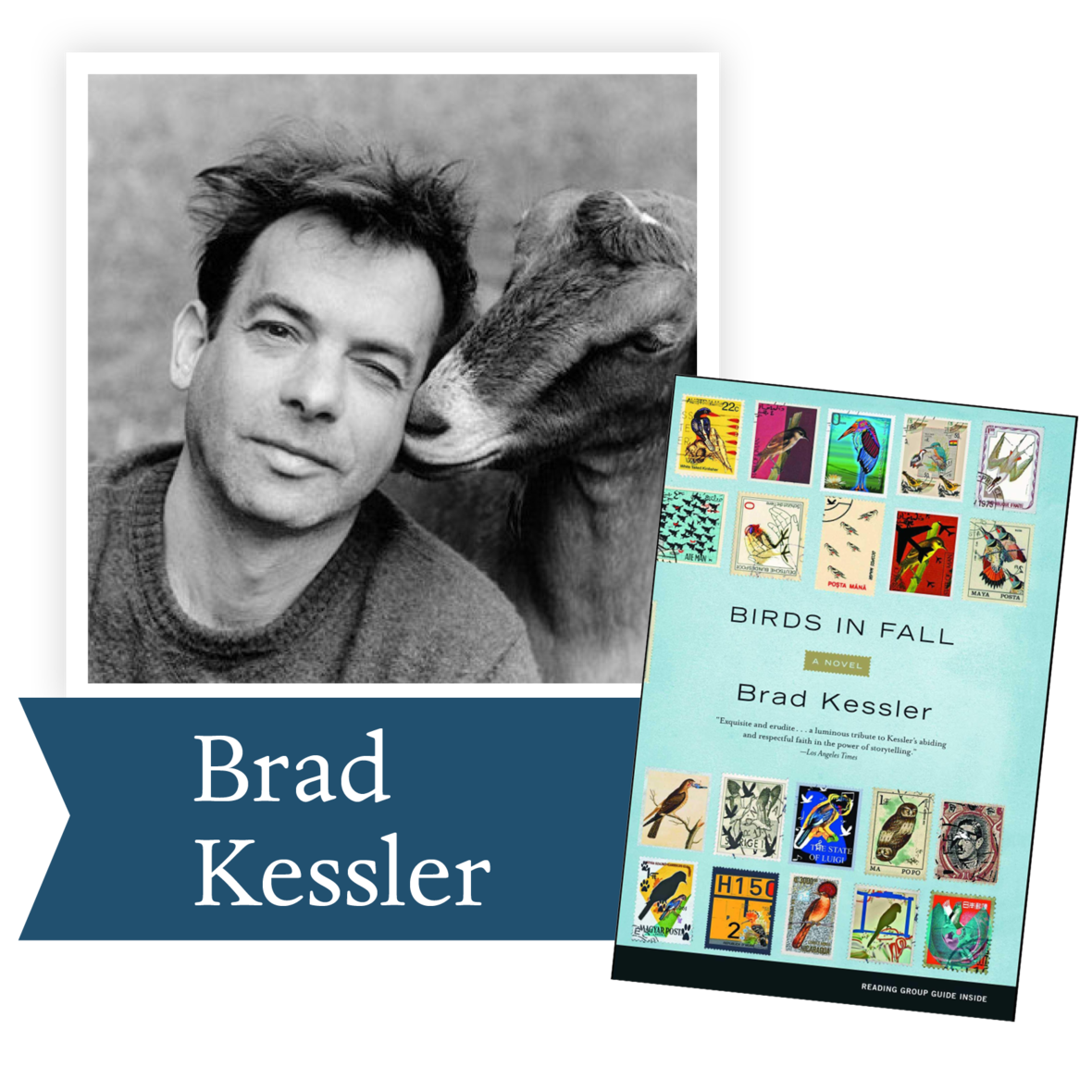Birds in Fall by Brad Kessler (Scribner): One fall night off the coast of a remote island in Nova Scotia, an airplane plummets to the sea as an innkeeper watches from the shore. Miles away in New York City, ornithologist Ana Gathreaux works in a darkened room, full of sparrows, testing their migratory instincts. Soon, Ana will be bound for Trachis Island, along with other relatives of victims who converge on the site of the tragedy.

 table div table+table+table+table+table+table+table+table+table+table+table+table+table+table+table+table div table{width:100%;padding:0}table div table+table+table+table+table+table+table+table+table+table+table+table+table+table+table+table div table img{width:96.23%;padding:0;float:none}table div table+table+table+table+table+table+table+table+table+table+table+table+table+table+table+table div table td{width:100%;padding:0 1.88% 18px}/* styles */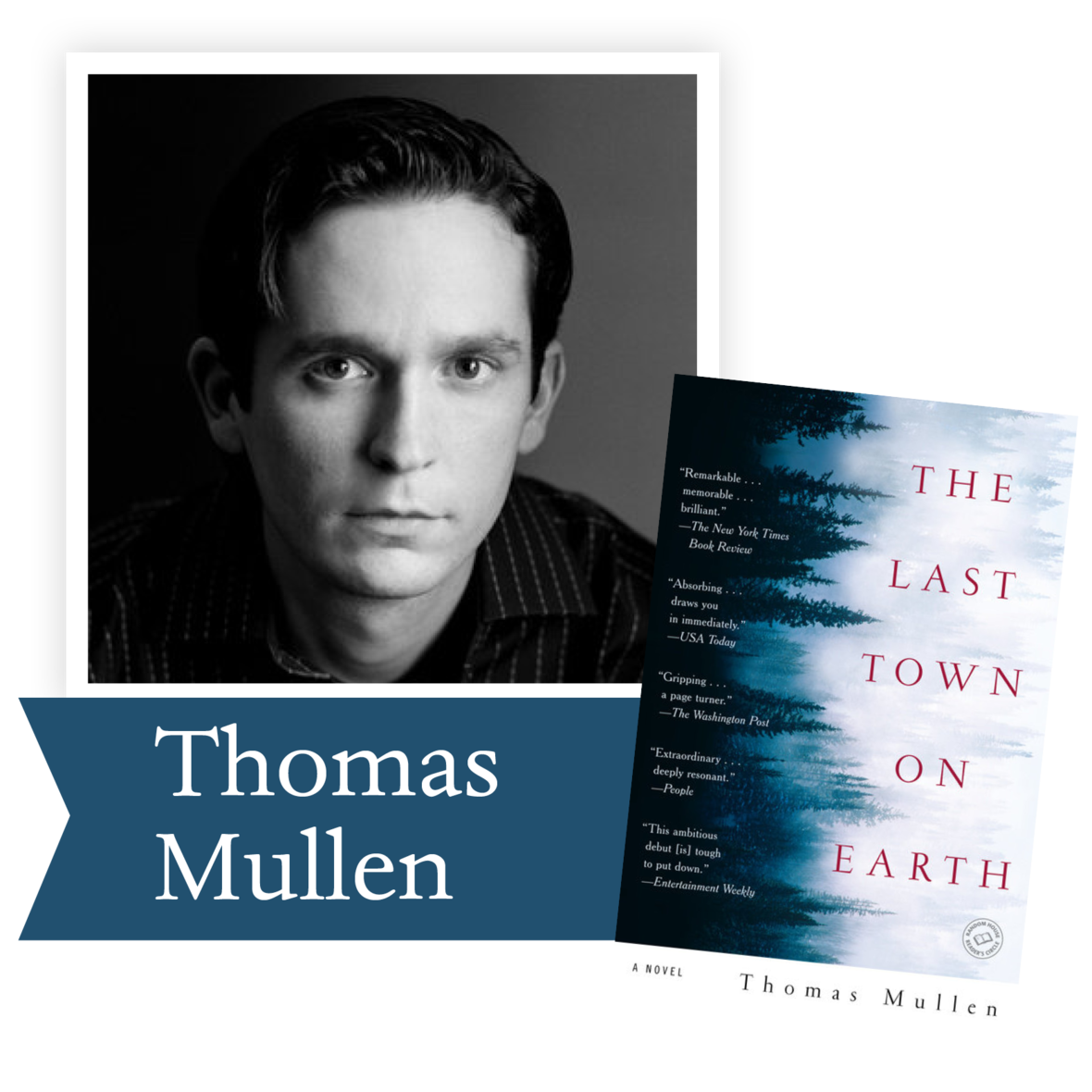The Last Town on Earth by Thomas Mullen (Random House): Set against the backdrop of one of the most virulent epidemics that America ever experienced–the 1918 flu epidemic–Thomas Mullen’s powerful, sweeping first novel is a tale of morality in a time of upheaval.

 table div table+table+table+table+table+table+table+table+table+table+table+table+table+table+table+table+table+table div table{width:100%;padding:0}table div table+table+table+table+table+table+table+table+table+table+table+table+table+table+table+table+table+table div table img{width:96.23%;padding:0;float:none}table div table+table+table+table+table+table+table+table+table+table+table+table+table+table+table+table+table+table div table td{width:100%;padding:0 1.88% 18px}/* styles */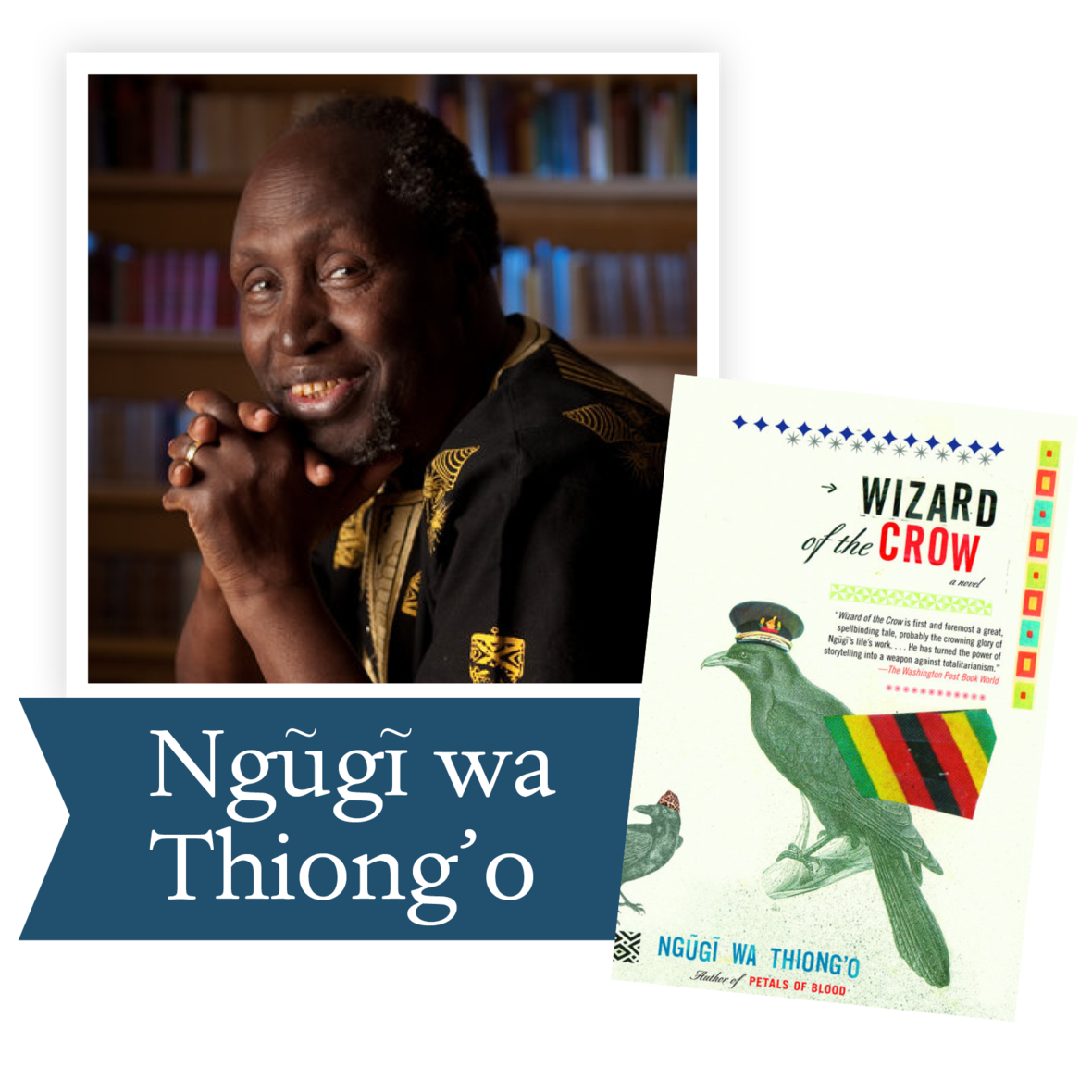The Wizard of the Crow by Ngugi wa’ Thiong’o (Pantheon): Set in the fictional Free Republic of Aburiria, Wizard of the Crow dramatizes with corrosive humor and keenness of observation a battle for the souls of the Aburirian people, between a megalomaniac dictator and an unemployed young man who embraces the mantle of a magician.

 table div table+table+table+table+table+table+table+table+table+table+table+table+table+table+table+table+table+table+table+table div table{width:100%;padding:0}table div table+table+table+table+table+table+table+table+table+table+table+table+table+table+table+table+table+table+table+table div table img{width:96.23%;padding:0;float:none}table div table+table+table+table+table+table+table+table+table+table+table+table+table+table+table+table+table+table+table+table div table td{width:100%;padding:0 1.88% 18px}/* styles */## 2007 Finalists - Nonfiction

 table div table+table+table+table+table+table+table+table+table+table+table+table+table+table+table+table+table+table+table+table+table+table div table{width:100%;padding:0}table div table+table+table+table+table+table+table+table+table+table+table+table+table+table+table+table+table+table+table+table+table+table div table img{width:96.23%;padding:0;float:none}table div table+table+table+table+table+table+table+table+table+table+table+table+table+table+table+table+table+table+table+table+table+table div table td{width:100%;padding:0 1.88% 18px}/* styles */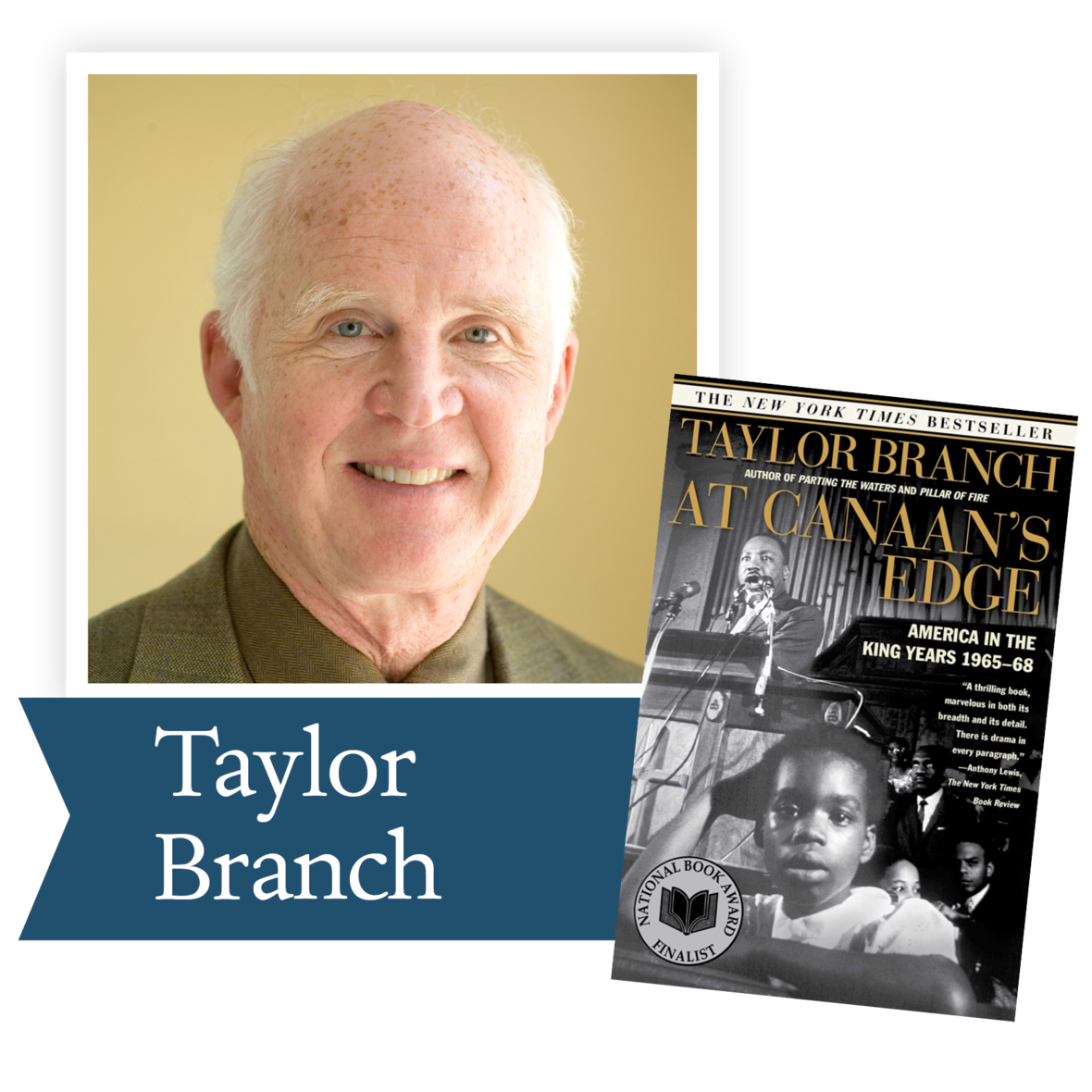At Canaan’s Edge by Taylor Branch (Simon and Schuster): At Canaan’s Edge concludes America in the King Years, a three-volume history that will endure as a masterpiece of storytelling on American race, violence, and democracy. Pulitzer Prize-winner and bestselling author Taylor Branch makes clear in this magisterial account of the civil rights movement that Martin Luther King, Jr., earned a place next to James Madison and Abraham Lincoln in the pantheon of American history.

 table div table+table+table+table+table+table+table+table+table+table+table+table+table+table+table+table+table+table+table+table+table+table+table+table div table{width:100%;padding:0}table div table+table+table+table+table+table+table+table+table+table+table+table+table+table+table+table+table+table+table+table+table+table+table+table div table img{width:96.23%;padding:0;float:none}table div table+table+table+table+table+table+table+table+table+table+table+table+table+table+table+table+table+table+table+table+table+table+table+table div table td{width:100%;padding:0 1.88% 18px}/* styles */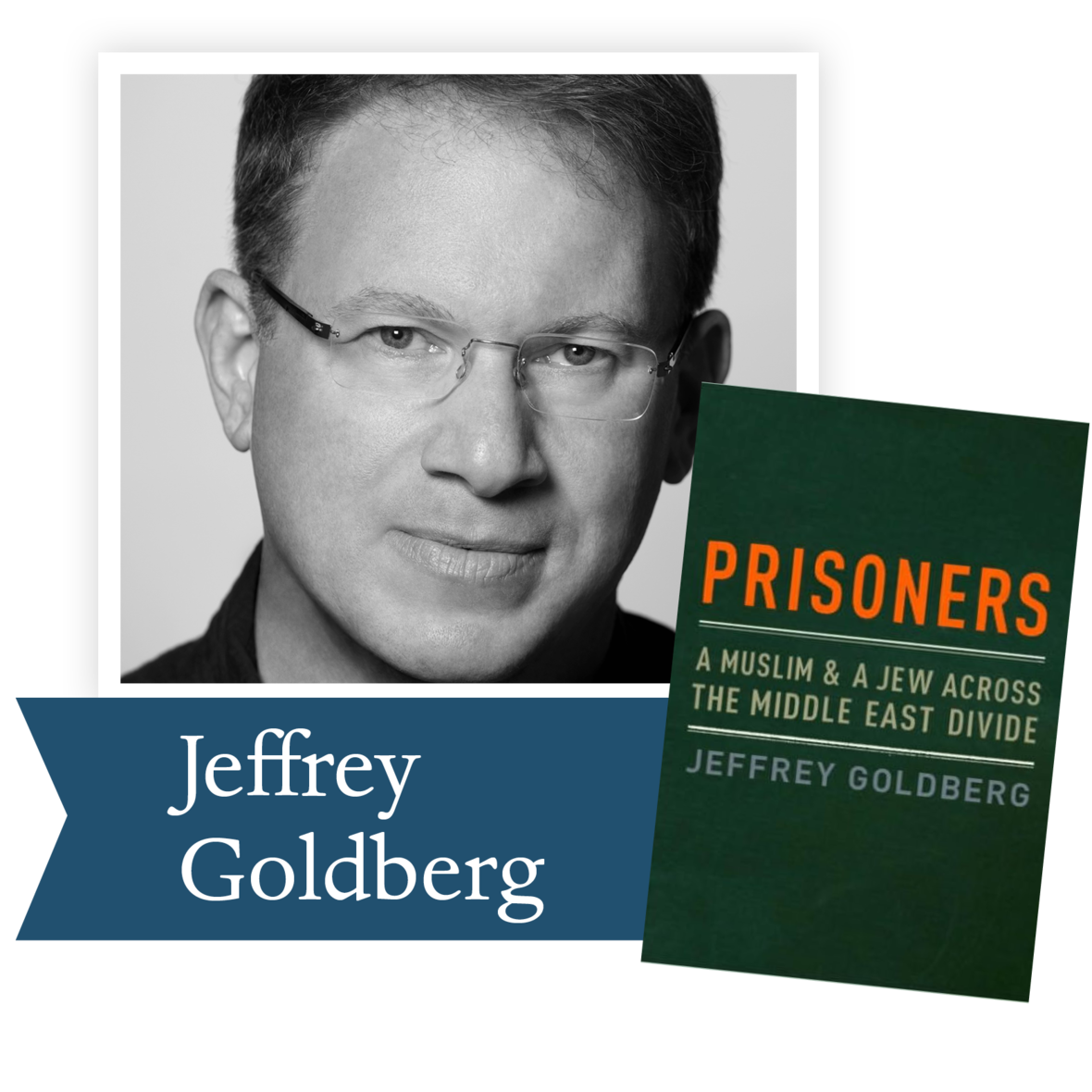Prisoners: A Muslim and a Jew Across the Continental Divide by Jeffrey Goldberg (Knopf): They met in 1990 during the first Palestinian uprising—one was an American Jew who served as a prison guard in the largest prison in Israel, the other, his prisoner, Rafiq, a rising leader in the PLO. Despite their fears and prejudices, they began a dialogue there that grew into a remarkable friendship—and now a remarkable book. It is a book that confronts head-on the issues dividing the Middle East, but one that also shines a ray of hope on that dark, embattled region.

 table div table+table+table+table+table+table+table+table+table+table+table+table+table+table+table+table+table+table+table+table+table+table+table+table+table+table div table{width:100%;padding:0}table div table+table+table+table+table+table+table+table+table+table+table+table+table+table+table+table+table+table+table+table+table+table+table+table+table+table div table img{width:96.23%;padding:0;float:none}table div table+table+table+table+table+table+table+table+table+table+table+table+table+table+table+table+table+table+table+table+table+table+table+table+table+table div table td{width:100%;padding:0 1.88% 18px}/* styles */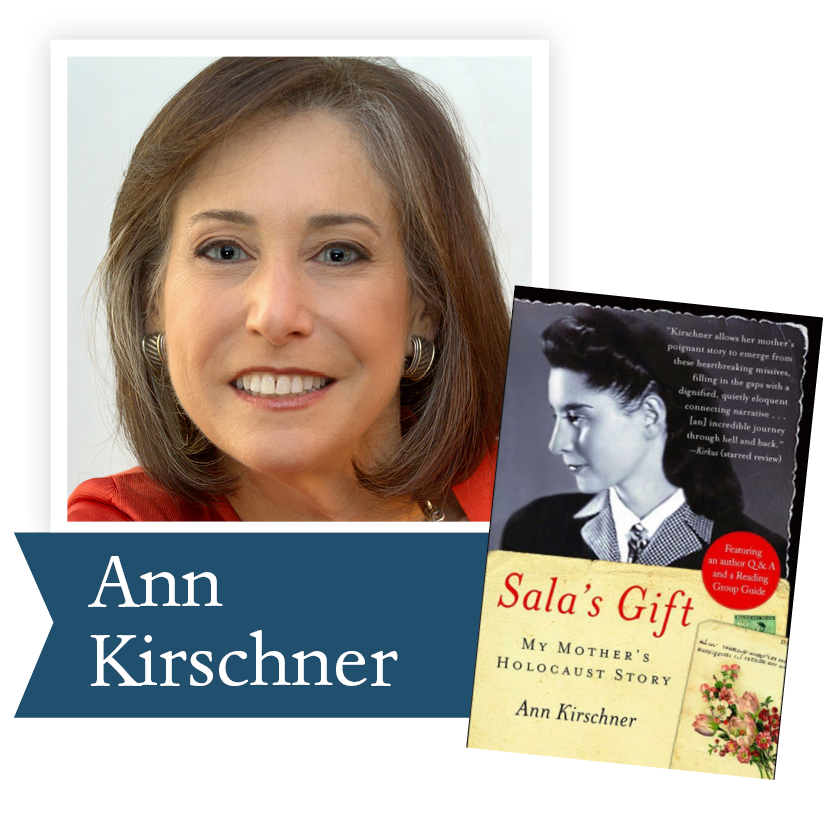Sala’s Gift by Ann Kirschner (Free Press): Ann Kirschner allows her mother's poignant story to emerge from these heartbreaking missives, filling in the gaps with a dignified, quietly eloquent connecting narrative…an incredible journey through hell and back.

 table div table+table+table+table+table+table+table+table+table+table+table+table+table+table+table+table+table+table+table+table+table+table+table+table+table+table+table+table div table{width:100%;padding:0}table div table+table+table+table+table+table+table+table+table+table+table+table+table+table+table+table+table+table+table+table+table+table+table+table+table+table+table+table div table img{width:96.23%;padding:0;float:none}table div table+table+table+table+table+table+table+table+table+table+table+table+table+table+table+table+table+table+table+table+table+table+table+table+table+table+table+table div table td{width:100%;padding:0 1.88% 18px}/* styles */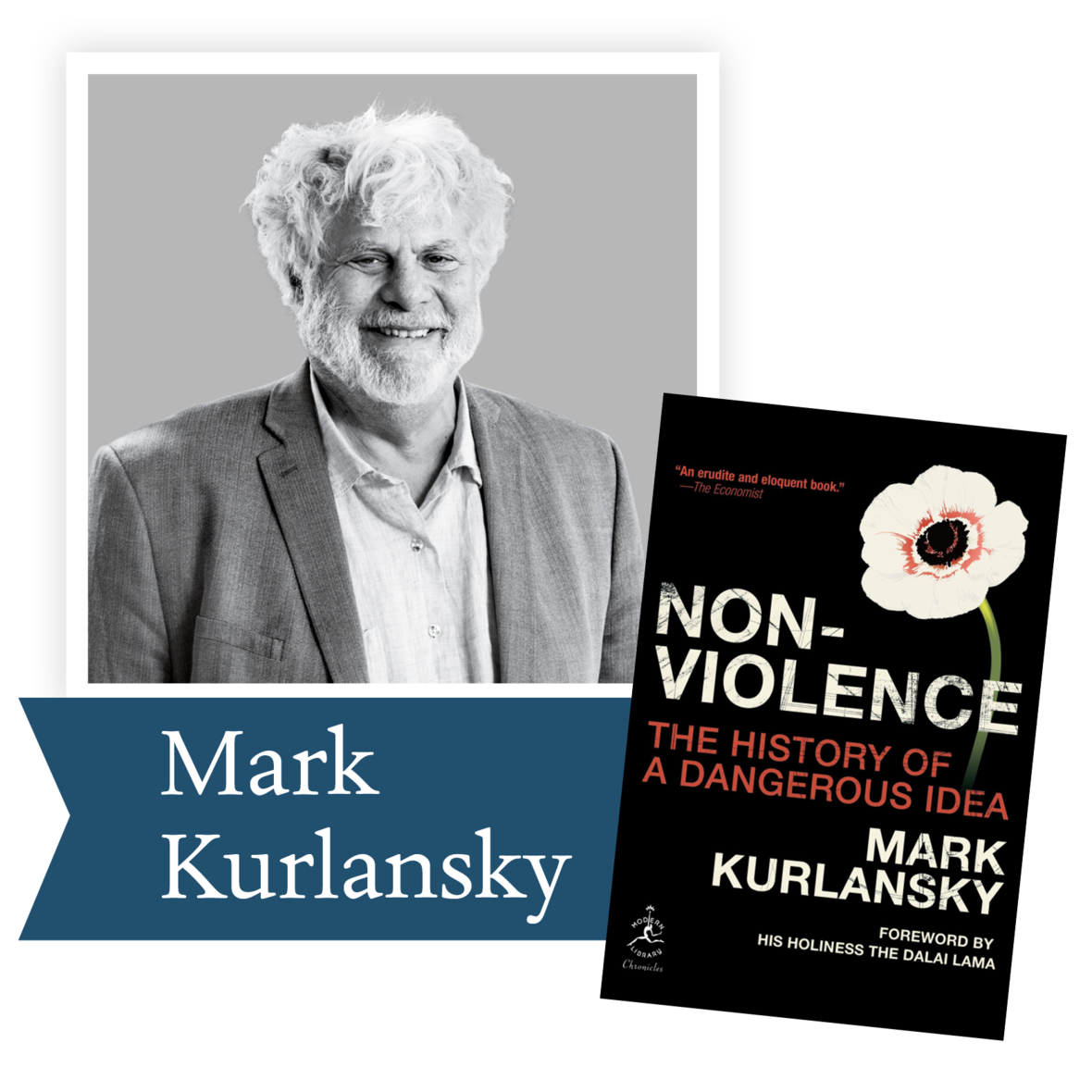Nonviolence: Twenty-five Lessons From The History of a Dangerous Idea by Mark Kurlansky (Modern Library): In this timely, highly original, and controversial narrative, author Mark Kurlansky discusses nonviolence as a course of action, rather than a mere state of mind: nonviolence can and should be a technique for overcoming social injustice and ending wars, which is why it is the preferred method of those who speak truth to power.

 table div table+table+table+table+table+table+table+table+table+table+table+table+table+table+table+table+table+table+table+table+table+table+table+table+table+table+table+table+table+table div table{width:100%;padding:0}table div table+table+table+table+table+table+table+table+table+table+table+table+table+table+table+table+table+table+table+table+table+table+table+table+table+table+table+table+table+table div table img{width:96.23%;padding:0;float:none}table div table+table+table+table+table+table+table+table+table+table+table+table+table+table+table+table+table+table+table+table+table+table+table+table+table+table+table+table+table+table div table td{width:100%;padding:0 1.88% 18px}/* styles */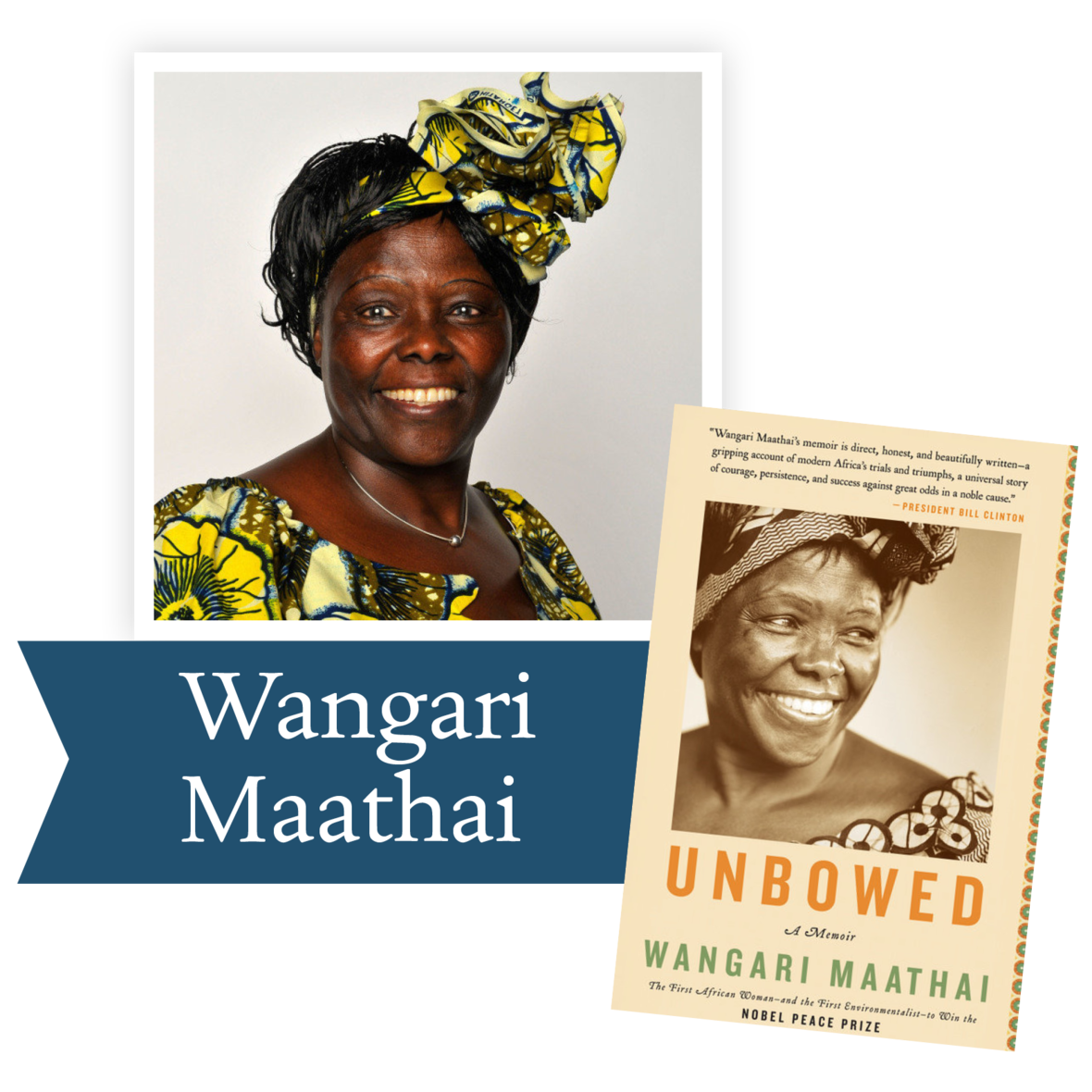Unbowed by Wangari Maathi (Anchor): Nobel Prize winner Wangari Maathai recounts her extraordinary journey from her childhood in rural Kenya to the world stage.

 table div table+table+table+table+table+table+table+table+table+table+table+table+table+table+table+table+table+table+table+table+table+table+table+table+table+table+table+table+table+table+table+table div table{width:100%;padding:0}table div table+table+table+table+table+table+table+table+table+table+table+table+table+table+table+table+table+table+table+table+table+table+table+table+table+table+table+table+table+table+table+table div table img{width:96.23%;padding:0;float:none}table div table+table+table+table+table+table+table+table+table+table+table+table+table+table+table+table+table+table+table+table+table+table+table+table+table+table+table+table+table+table+table+table div table td{width:100%;padding:0 1.88% 18px}/* styles */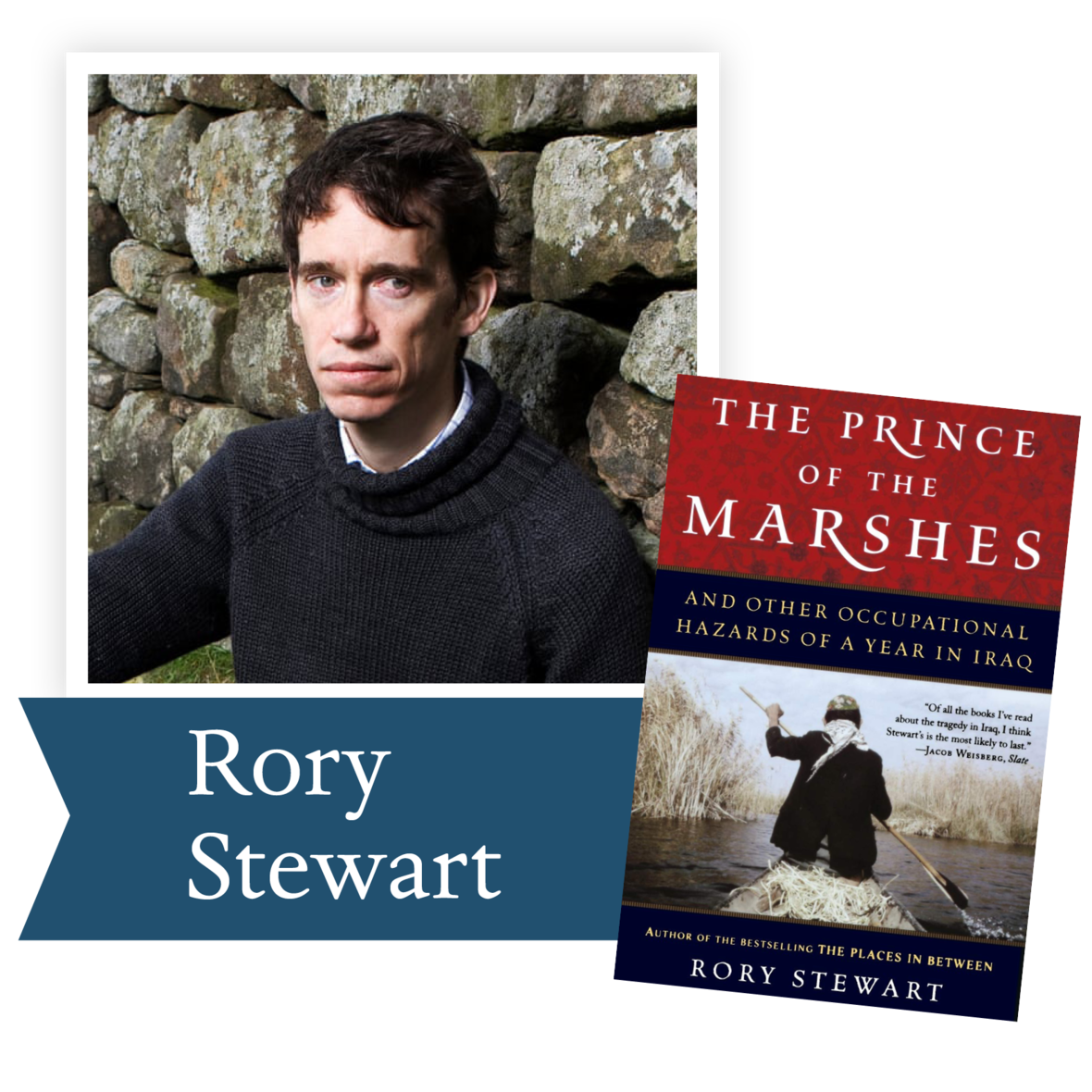Prince of the Marshes by Rory Stewart (Houghton Mifflin Company): In August 2003, at the age of thirty, Rory Stewart took a taxi from Jordan to Baghdad. A Farsi-speaking British diplomat who had recently completed an epic walk from Turkey to Bangladesh, he was soon appointed deputy governor of Amarah and then Nasiriyah, provinces in the remote, impoverished marsh regions of southern Iraq. He spent the next eleven months negotiating hostage releases, holding elections, and splicing together some semblance of an infrastructure for a population of millions teetering on the brink of civil war.

 table div table+table+table+table+table+table+table+table+table+table+table+table+table+table+table+table+table+table+table+table+table+table+table+table+table+table+table+table+table+table+table+table+table+table div table{width:100%;padding:0}table div table+table+table+table+table+table+table+table+table+table+table+table+table+table+table+table+table+table+table+table+table+table+table+table+table+table+table+table+table+table+table+table+table+table div table img{width:96.23%;padding:0;float:none}table div table+table+table+table+table+table+table+table+table+table+table+table+table+table+table+table+table+table+table+table+table+table+table+table+table+table+table+table+table+table+table+table+table+table div table td{width:100%;padding:0 1.88% 18px}/* styles */## 2007 Lifetime Achievement Award Winner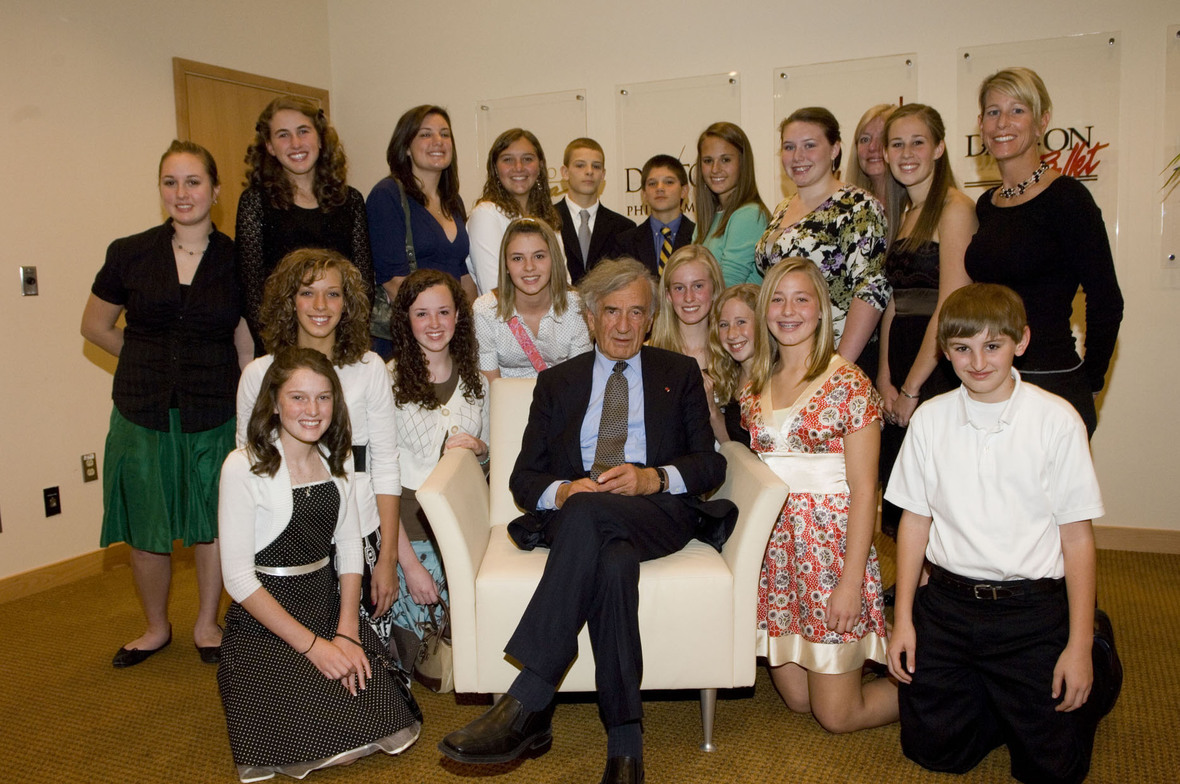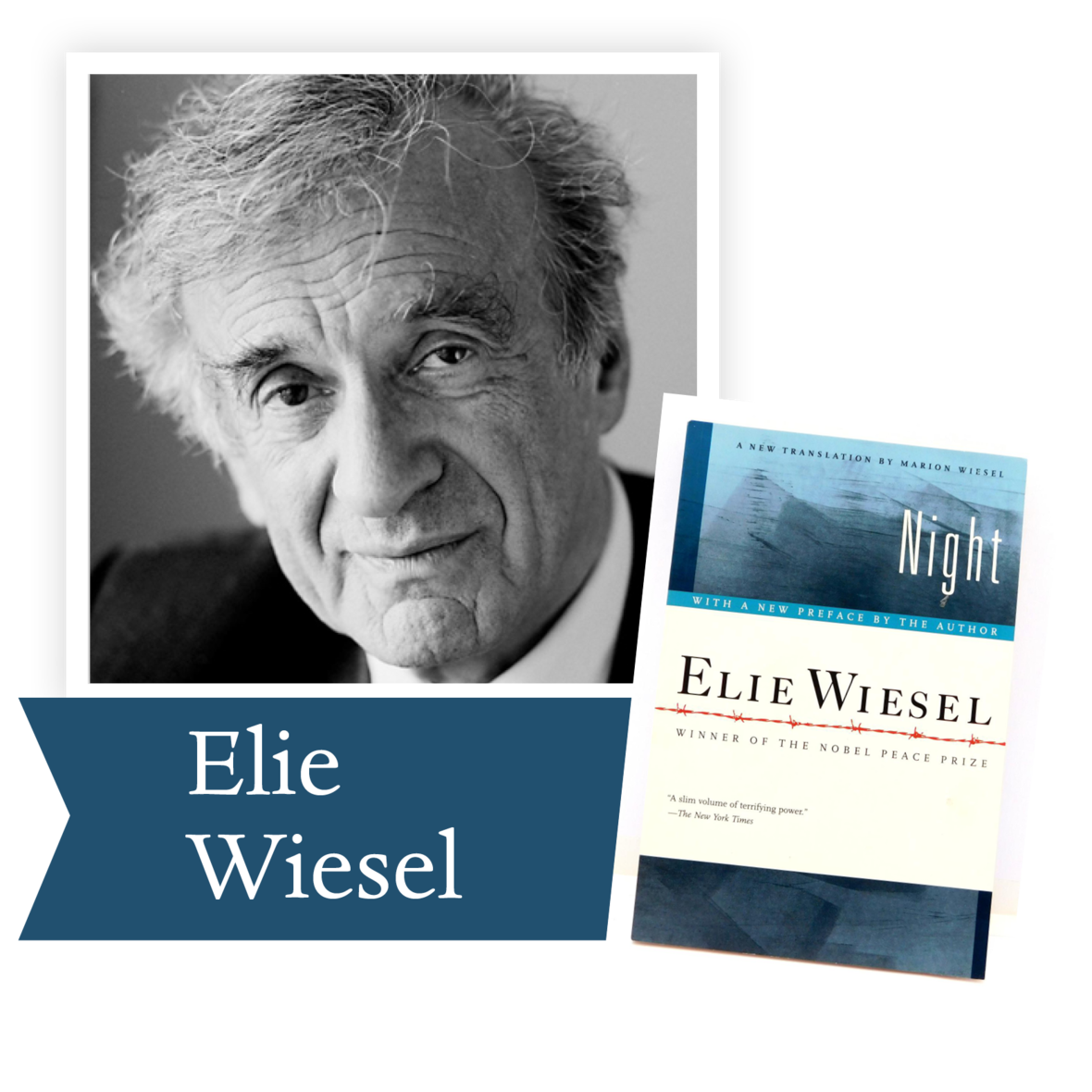Elie Wiesel’s Night is keystone of the cannon of Holocaust literature read by students throughout the world. In little more than one hundred pages, Wiesel describes his imprisonment with his father in Auschwitz and Buchenwald in 1944-1945. Wiesel was fifteen at the time, which may explain why he spent most of the evening prior to the 2007 DLPP awards ceremony talking with the students DLPP had invited to come to the Schuster Center to hear him speak. He spent far more time with them than we anticipated, quietly and thoughtfully answering their questions, listening to their comments, signing their books, shaking their hands, spending a private moment with each of them. It was a special evening in DLPP history.

Many adults have not had the experience of reading this book, nor the others in his Holocaust trilogy, Dawn and Day. We invite you to read them to see why the students revered their time with this man of peace.

 table div table+table+table+table+table+table+table+table+table+table+table+table+table+table+table+table+table+table+table+table+table+table+table+table+table+table+table+table+table+table+table+table+table+table+table+table+table+table div table{width:100%;padding:0}table div table+table+table+table+table+table+table+table+table+table+table+table+table+table+table+table+table+table+table+table+table+table+table+table+table+table+table+table+table+table+table+table+table+table+table+table+table+table div table img{width:96.23%;padding:0;float:none}table div table+table+table+table+table+table+table+table+table+table+table+table+table+table+table+table+table+table+table+table+table+table+table+table+table+table+table+table+table+table+table+table+table+table+table+table+table+table div table td{width:100%;padding:0 1.88% 18px}/* styles */table div table+table+table+table+table+table+table+table+table+table+table+table+table+table+table+table+table+table+table+table+table+table+table+table+table+table+table+table+table+table+table+table+table+table+table+table+table+table+table+table div table{width:100%;padding:0}table div table+table+table+table+table+table+table+table+table+table+table+table+table+table+table+table+table+table+table+table+table+table+table+table+table+table+table+table+table+table+table+table+table+table+table+table+table+table+table+table div table img{width:96.23%;padding:0;float:none}table div table+table+table+table+table+table+table+table+table+table+table+table+table+table+table+table+table+table+table+table+table+table+table+table+table+table+table+table+table+table+table+table+table+table+table+table+table+table+table+table div table td{width:100%;padding:0 1.88% 18px}/* styles */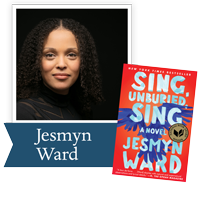Jesmyn Ward, a three-time finalist for the Dayton Literary Peace Prize, won the National Book Award for Sing, Unburied, Sing. Her work can educate us about the racial issues facing America today.

“The heart of Jesmyn Ward’s Sing, Unburied, Sing is story—the yearning for a narrative to help us understand ourselves, the pain of the gaps we’ll never fill, the truths that are failed by words and must be translated through ritual and song...Ward’s writing throbs with life, grief, and love, and this book is the kind that makes you ache to return to it.” —Buzzfeed

Ron Rollins, recently retired Dayton Daily News Ideas and Voices Editor, will moderate the discussion.

 table div table+table+table+table+table+table+table+table+table+table+table+table+table+table+table+table+table+table+table+table+table+table+table+table+table+table+table+table+table+table+table+table+table+table+table+table+table+table+table+table+table+table div table{width:100%;padding:0}table div table+table+table+table+table+table+table+table+table+table+table+table+table+table+table+table+table+table+table+table+table+table+table+table+table+table+table+table+table+table+table+table+table+table+table+table+table+table+table+table+table+table div table img{width:96.23%;padding:0;float:none}table div table+table+table+table+table+table+table+table+table+table+table+table+table+table+table+table+table+table+table+table+table+table+table+table+table+table+table+table+table+table+table+table+table+table+table+table+table+table+table+table+table+table div table td{width:100%;padding:0 1.88% 18px}/* styles */